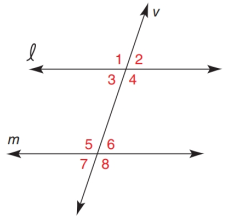Chapter 2.1, Problem 2E### Elementary Geometry for College St...

6th Edition
Daniel C. Alexander + 1 other
ISBN: 9781285195698

#### Solutions

Chapter
Section### Elementary Geometry for College St...

6th Edition
Daniel C. Alexander + 1 other
ISBN: 9781285195698
Textbook Problem
1 views

# For Exercises 1 to 4, l ∥ m with transversal v .If m ∠ 3 = 71 ° , find: a) m ∠ 5 b) m ∠ 6Exercises 1 − 4

To determine

a)

To find:

The value of m5.

Explanation

Given:

The given figure is,

𝓁m with transversal v and m3=71°.

Definition:

The pairs of angles on one side of the transversal but inside the two lines are called Consecutive Interior Angles. Sum of measures of these angles is 180°.

Calculation:

Consider the given figure,

Figure (1)

From the figure (1), 𝓁m with transversal v.

3 and 5 are forming Consecutive interior angles

To determine

b)

To find:

The value of m6.

### Still sussing out bartleby?

Check out a sample textbook solution.

See a sample solution

#### The Solution to Your Study Problems

Bartleby provides explanations to thousands of textbook problems written by our experts, many with advanced degrees!

Get Started

#### Multiply: (3)(9)

Elementary Technical Mathematics

#### In Problems 1-12, find the derivative of each function. 9.

Mathematical Applications for the Management, Life, and Social Sciences

#### limh0ah1h=1 for a = a) 0 b) 1 c) e d) The limit is never 1.

Study Guide for Stewart's Single Variable Calculus: Early Transcendentals, 8th